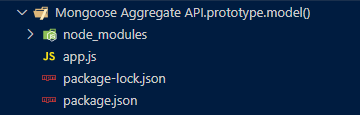Open in App
Not now

# Mongoose Aggregate.prototype.model() API

• Last Updated : 30 Dec, 2022

The Mongoose Aggregate prototype.model() method of the Mongoose API is used to perform aggregation tasks. It allows us to change the model for any particular aggregation pipeline. This method accepts the model object to return the model object for that particular aggregation pipeline. Let us understand model() method using an example.

Syntax:

`aggregate.model( model_name );`

Parameters: This method accepts a single parameter as discussed below:

• model: This method takes a model object as a parameter.

Return Value: This method returns the model object on which the aggregation pipeline will get executed.

Setting up Node.js application:

Step 1: Create a Node.js application using the following command:

`npm init`

Step 2: After creating the NodeJS application, Install the required module using the following command:

`npm install mongoose`

Project Structure: The project structure will look like this:Example 1: In this example, we are illustrating the functionality of model() method by defining the aggregate pipeline on the Student model.

Filename: app.js

## Javascript

 `// Require mongoose module ` `const mongoose = require(``"mongoose"``); ` ` `  `// Set Up the Database connection ` `const URI = ``"mongodb://localhost:27017/geeksforgeeks"` ` `  `const connectionObject = mongoose.createConnection(URI, { ` `    ``useNewUrlParser: ``true``, ` `    ``useUnifiedTopology: ``true``, ` `}); ` ` `  `const studentSchema = ``new` `mongoose.Schema({ ` `    ``name: { type: String, required: ``true` `}, ` `    ``age: { type: Number, required: ``false` `}, ` `    ``rollNumber: { type: Number }, ` `}); ` ` `  `const Student =  ` `    ``connectionObject.model(``'Student'``, studentSchema); ` ` `  `const aggregate =  ` `    ``Student.aggregate([{ \$project: { age: 1 } }]) ` `     `  `console.log(aggregate.model() === Student);`

Step to run the program: To run the application execute the below command from the root directory of the project:

`node app.js`

Output:

`true`

Example 2: In this example, we are illustrating the functionality of model() method by passing Customer model to the aggregate object returned by the Student model aggregate pipeline.

Filename: app.js

## Javascript

 `// Require mongoose module ` `const mongoose = require(``"mongoose"``); ` ` `  `// Set Up the Database connection ` `const URI = ``"mongodb://localhost:27017/geeksforgeeks"` ` `  `const connectionObject = mongoose.createConnection(URI, { ` `    ``useNewUrlParser: ``true``, ` `    ``useUnifiedTopology: ``true``, ` `}); ` ` `  `const studentSchema = ``new` `mongoose.Schema({ ` `    ``name: { type: String, required: ``true` `}, ` `    ``age: { type: Number, required: ``false` `}, ` `    ``rollNumber: { type: Number }, ` `}); ` ` `  `const Student = connectionObject.model(``'Student'``, studentSchema); ` ` `  `const Customer = connectionObject.model( ` `    ``'Customer'``, ``new` `mongoose.Schema({ ` `        ``name: String, ` `        ``address: String, ` `        ``orderNumber: Number, ` `    ``})); ` ` `  `const aggregate = Student.aggregate([{ \$project: { age: 1 } }]) ` ` `  `aggregate.model(Customer); ` ` `  `console.log(aggregate.model() === Customer);`

Step to run the program: To run the application execute the below command from the root directory of the project:

`node app.js`

Output:

`false`

My Personal Notes arrow_drop_up
Related Articles